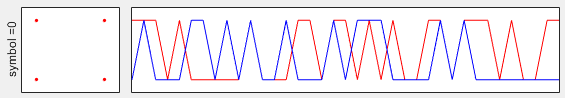5G - Pre Trial - Physical Signal - BRRS                    Home : www.sharetechnote.com

BRRS (Beam Refinement Reference Signal)

 Parameter BRRS NID_BRRS = 0; ns = 0; l = 0;Disclaimer : This code is just to push myself (probably readers) to look into the algorithm (formula) specified in the specification to the most detailed level. If you try to convert the specification into the programming code whatever language you choose, you will understand the equation / algorithm in much more detailed level than just reading the document. However, this code has not been verified with any real data.

 Filename : Generate_Brrs.m Last Update : Dec 25, 2016 %V5G.211 - 6.7.5.1 function SequenceBrrs = Generate_Brrs(BRRS)       NID_BRRS = BRRS.NID_BRRS;     N_RB_MAX_DL = BRRS.N_RB_MAX_DL;     ns = BRRS.ns;     l = BRRS.Symbol;     ns_bar = mod(BRRS.ns,20);          r_m = [];          % Generate Psuedo Random Sequence     C_init = 2^10 * (7*(ns_bar + 1) + l + 1) * (2 * NID_BRRS + 1) + 2 * NID_BRRS + 1;       r_m_l = [];     c_m_even = [];     c_m_odd = [];       PR.x2_init = C_init;       for m = 0 : (floor(3/8 * N_RB_MAX_DL)-1)       PR.n = 2*m;       c_m = Generate_PR(PR);       c_m_even = [c_m_even c_m];       PR.n = 2*m+1;       c_m = Generate_PR(PR);       c_m_odd = [c_m_odd c_m];     end;       r_m_l = (1 ./ sqrt(2) * (1 - 2 .* c_m_even)) + (j .* 1 ./ sqrt(2) * (1 - 2 .* c_m_odd));           SequenceBrrs.r_m_l = r_m_l;      end

 Filename : PlotSequence_Brrs.m Last Update : Dec 25, 2016 function h=PlotSequence_Brrs(BrrsSequence,PlotOption)       plotData = BrrsSequence.r_m_l;     if isfield(PlotOption,'NumOfDataToPlot') == 1        NumOfDataToPlot = PlotOption.NumOfDataToPlot;     end;          l = PlotOption.Symbol;     w = 10;           d_n=plotData;       subplot(1,w,[1 (l*w)+2]);     plot(real(d_n),imag(d_n),'ro', ...          'MarkerFaceColor',[1 0 0],'MarkerSize',2);     set(gca,'xticklabel',[]);set(gca,'yticklabel',[]);     set(gca,'xtick',[]);set(gca,'ytick',[]);     ylabel(strcat('symbol = ',num2str(l)));     set(gca,'fontsize',8);       subplot(1,w,[((l*w)+3) ((l*w)+w)]);     n = 0:length(d_n)-1;     plot(n,real(d_n),'r-',n,imag(d_n),'b-');     if exist('NumOfDataToPlot') == 1         xlim([0 NumOfDataToPlot]);     else             xlim([0 n(end)]);     end;         set(gca,'xticklabel',[]);set(gca,'yticklabel',[]);     set(gca,'xtick',[]);set(gca,'ytick',[]);      end

 Filename : Test_Generation_Brrs.m Last Update : Dec 25, 2016 clear PlotOption = [];   BRRS.NID_BRRS = 0; BRRS.N_RB_MAX_DL = 100; BRRS.ns = 0; BRRS.Symbol = 0;   BrrsSequence = Generate_Brrs(BRRS);   %PlotOption.NumOfDataToPlot = 16; PlotOption.Symbol = 0; PlotSequence_Brrs(BrrsSequence,PlotOption);